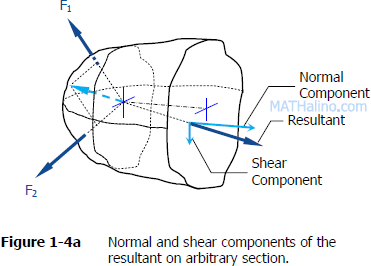## Solution to Problem 249 Statically Indeterminate

Problem 249
There is a radial clearance of 0.05 mm when a steel tube is placed over an aluminum tube. The inside diameter of the aluminum tube is 120 mm, and the wall thickness of each tube is 2.5 mm. Compute the contact pressure and tangential stress in each tube when the aluminum tube is subjected to an internal pressure of 5.0 MPa.

## Solution to Problem 142 Pressure Vessel

Problem 142
A pipe carrying steam at 3.5 MPa has an outside diameter of 450 mm and a wall thickness of 10 mm. A gasket is inserted between the flange at one end of the pipe and a flat plate used to cap the end. How many 40-mm-diameter bolts must be used to hold the cap on if the allowable stress in the bolts is 80 MPa, of which 55 MPa is the initial stress? What circumferential stress is developed in the pipe? Why is it necessary to tighten the bolt initially, and what will happen if the steam pressure should cause the stress in the bolts to be twice the value of the initial stress?

## Solution to Problem 138 Pressure Vessel

Problem 138
The strength of longitudinal joint in Fig. 1-17 is 33 kips/ft, whereas for the girth is 16 kips/ft. Calculate the maximum diameter of the cylinder tank if the internal pressure is 150 psi.

## Solution to Problem 137 Pressure Vessel

Problem 137
A water tank, 22 ft in diameter, is made from steel plates that are 1/2 in. thick. Find the maximum height to which the tank may be filled if the circumferential stress is limited to 6000 psi. The specific weight of water is 62.4 lb/ft3.

## Solution to Problem 136 Pressure Vessel

Problem 136
A cylindrical pressure vessel is fabricated from steel plating that has a thickness of 20 mm. The diameter of the pressure vessel is 450 mm and its length is 2.0 m. Determine the maximum internal pressure that can be applied if the longitudinal stress is limited to 140 MPa, and the circumferential stress is limited to 60 MPa.

## Solution to Problem 135 Pressure Vessel

Problem 135
Calculate the minimum wall thickness for a cylindrical vessel that is to carry a gas at a pressure of 1400 psi. The diameter of the vessel is 2 ft, and the stress is limited to 12 ksi.

## Solution to Problem 133 Pressure Vessel

Problem 133
A cylindrical steel pressure vessel 400 mm in diameter with a wall thickness of 20 mm, is subjected to an internal pressure of 4.5 MN/m2. (a) Calculate the tangential and longitudinal stresses in the steel. (b) To what value may the internal pressure be increased if the stress in the steel is limited to 120 MN/m2? (c) If the internal pressure were increased until the vessel burst, sketch the type of fracture that would occur.

## Solution to Problem 122 Shear Stress

Problem 122
Two blocks of wood, width w and thickness t, are glued together along the joint inclined at the angle θ as shown in Fig. P-122. Using the free-body diagram concept in Fig. 1-4a, show that the shearing stress on the glued joint is τ = P sin 2θ / 2A, where A is the cross-sectional area.